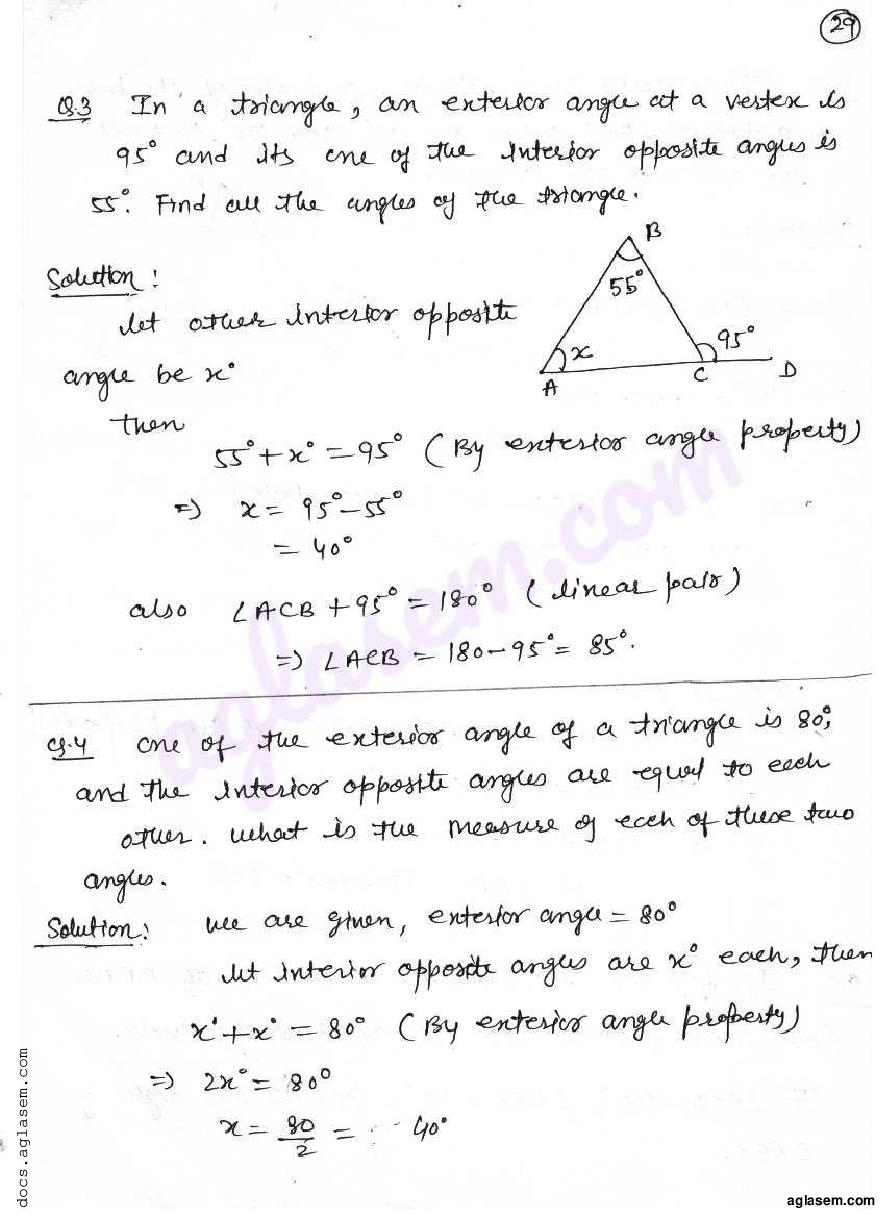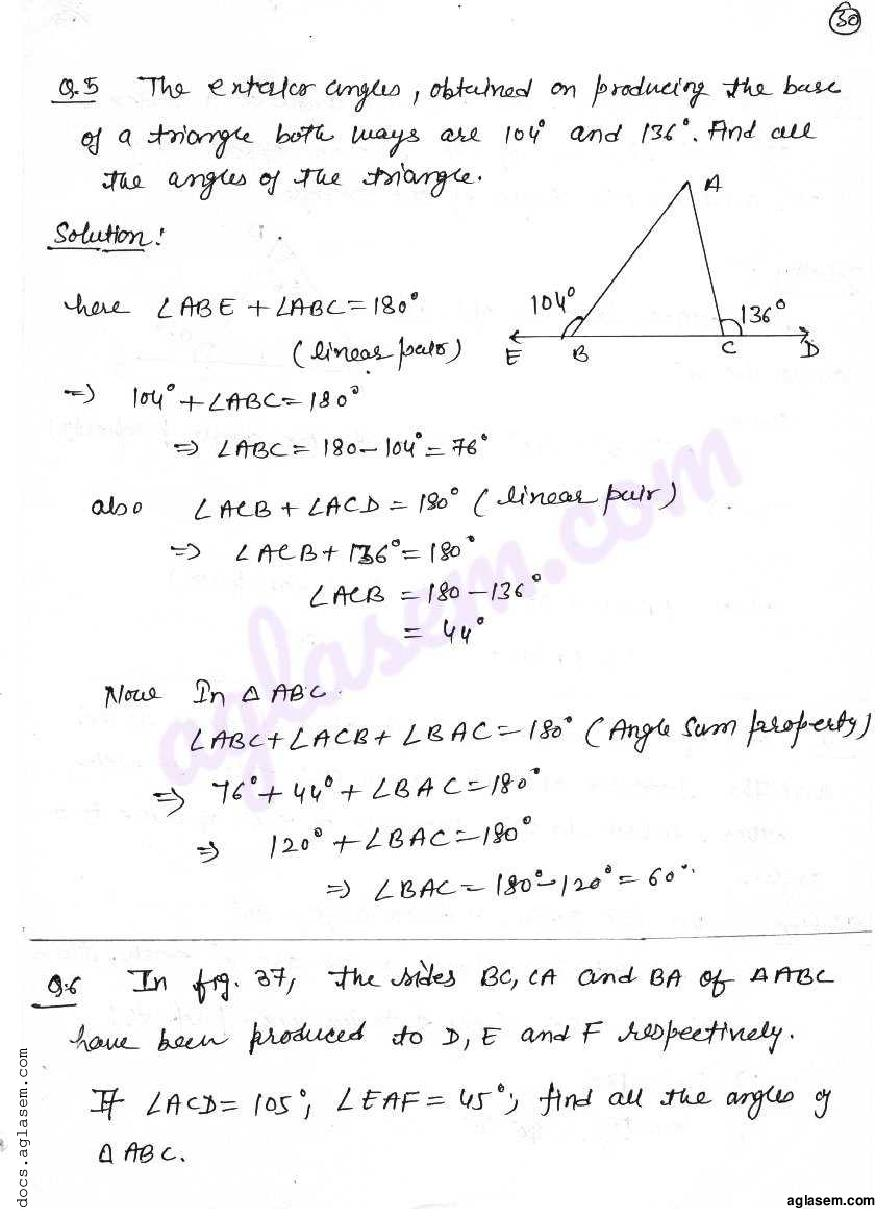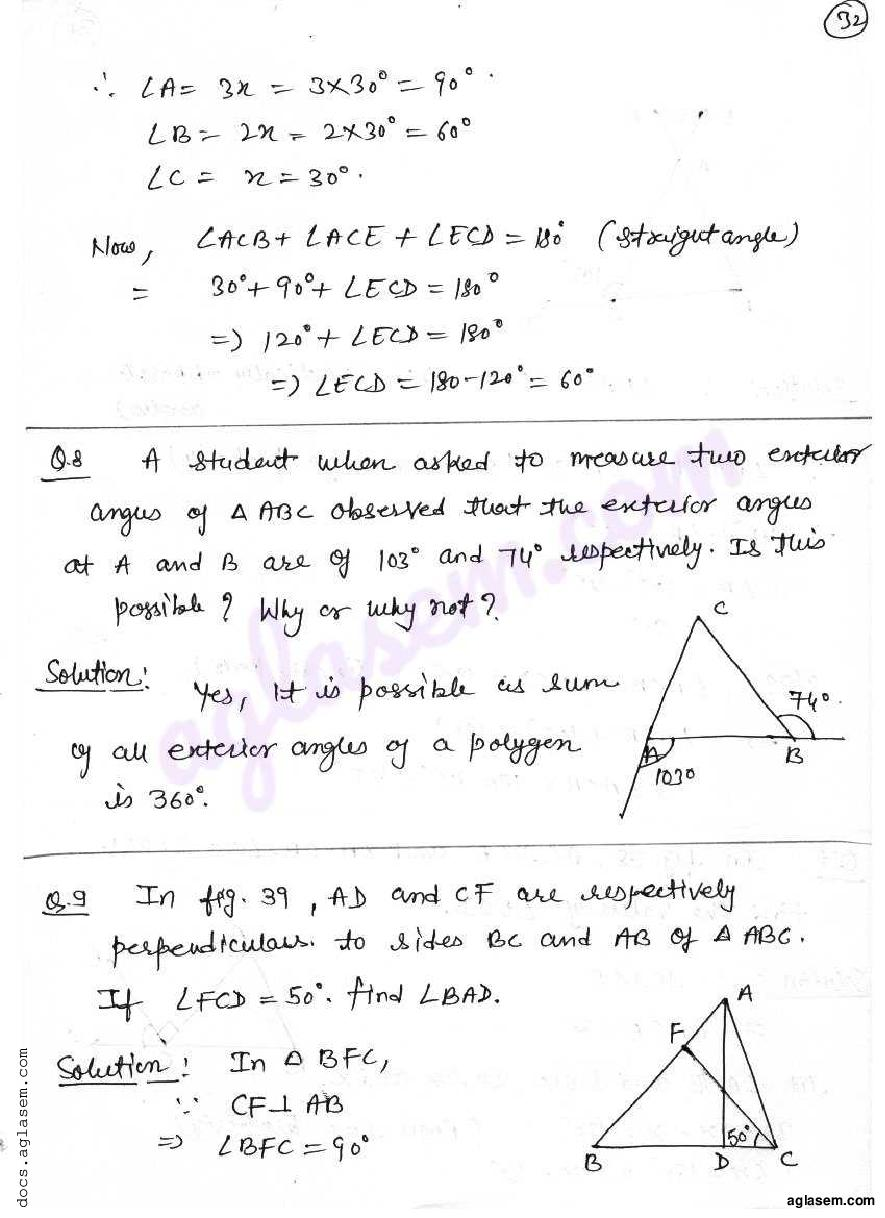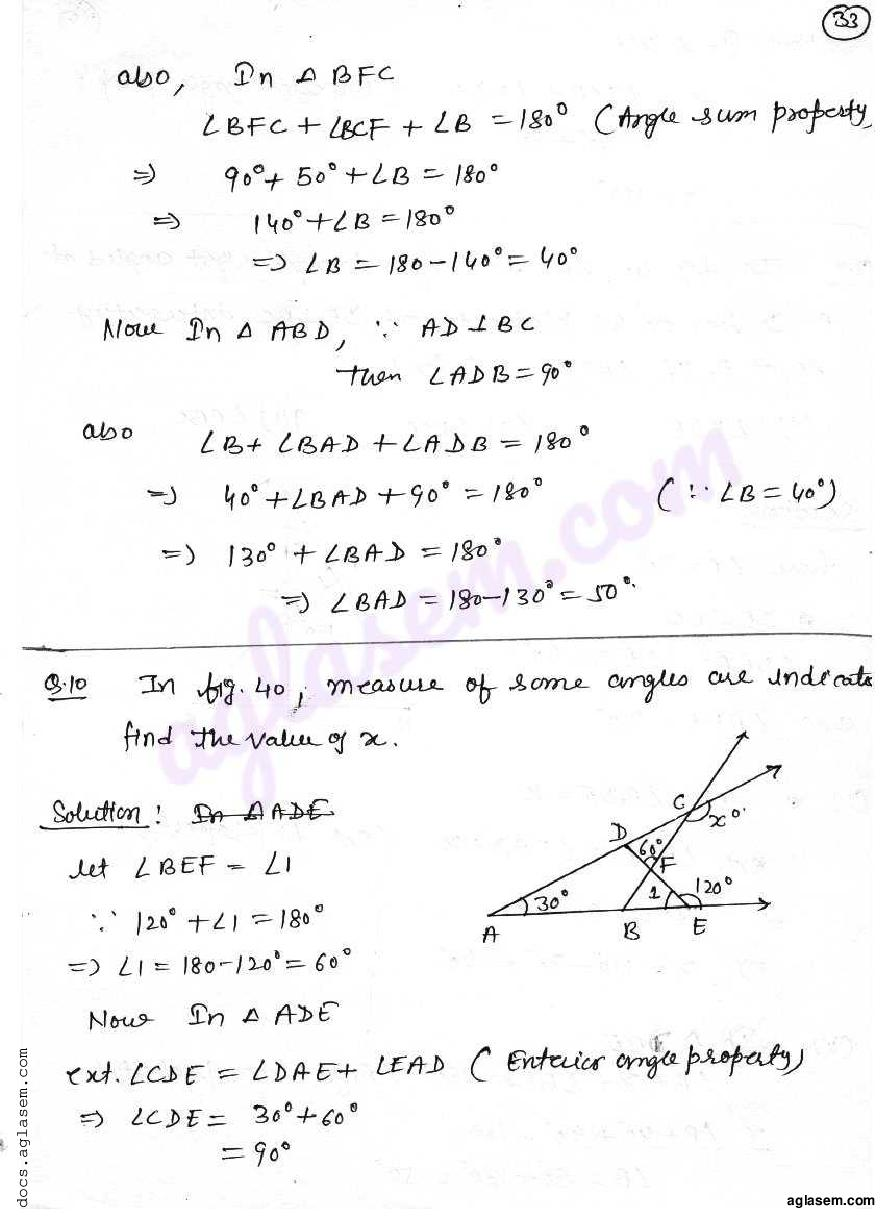# RD Sharma Solutions Class 7 Chapter 15 Properties of Triangles Exercise 15.3

Here you can get free RD Sharma Solutions for Class 7 Maths Chapter 15 Properties of Triangles Exercise 15.3. All RD Sharma Book Solutions are given here exercise wise for the chapter Properties of Triangles. RD Sharma Solutions are helpful in the preparation of several school level, graduate and undergraduate level competitive exams. Practicing questions from RD Sharma Mathematics Solutions for Class 7 Chapter 15 Properties of Triangles is proven to enhance your math skills.

 Class: Class 7th Chapter: Chapter 15 Exercise: Exercise 15.3 Name: Properties of Triangles

## RD Sharma Solutions Class 7 Chapter 15 Properties of Triangles Exercise 15.3

RD Sharma Class 7 Solutions Chapter 15 for Properties of Triangles Exercise 15.3 are given below.

RD Sharma Solutions Class 7 Chapter 15 Properties of Triangles Exercise 15.3